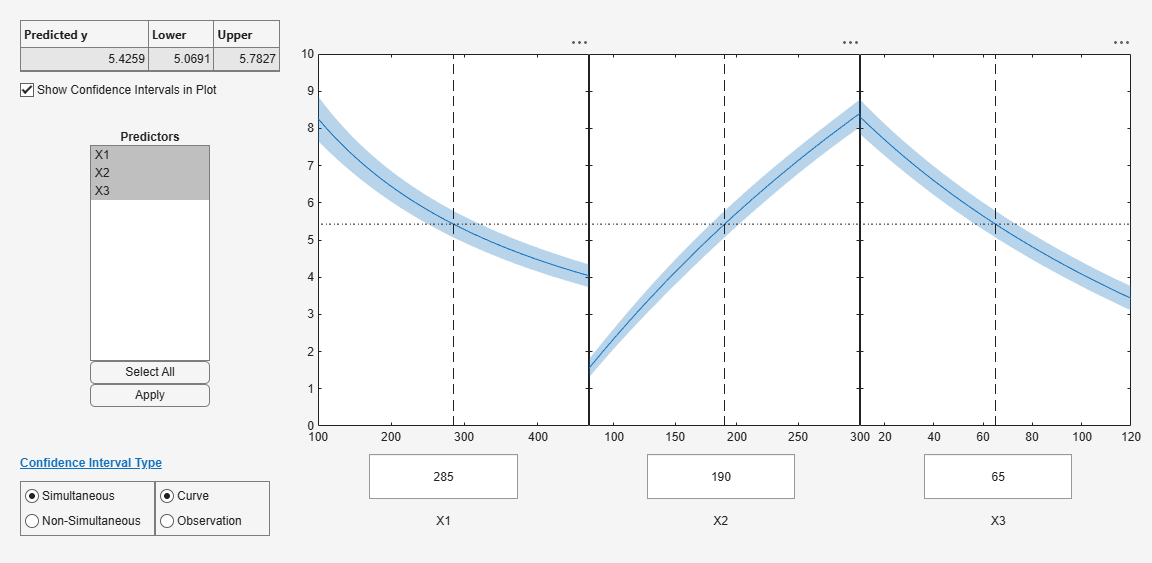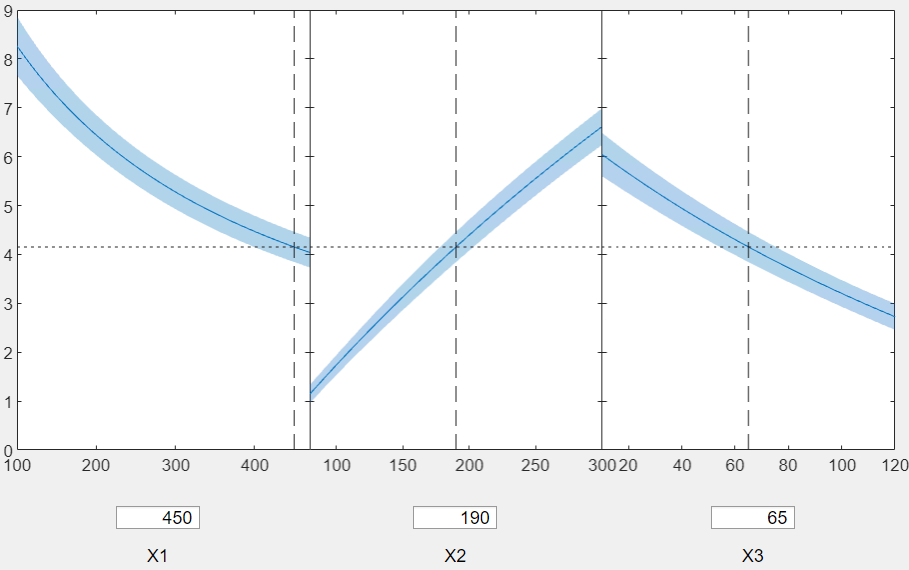# plotSlice

Class: NonLinearModel

Plot of slices through fitted nonlinear regression surface

## Syntax

```plotSlice(mdl) ```

## Description

`plotSlice(mdl)` creates a new figure containing a series of plots, each representing a slice through the regression surface predicted by `mdl`. For each plot, the surface slice is shown as a function of a single predictor variable, with the other predictor variables held constant.

## Input Arguments

 `mdl` Nonlinear regression model, constructed by `fitnlm`.

## Examples

expand all

Plot slices of a fitted nonlinear model.

Load the `reaction` data and fit a model of the reaction rate as a function of reactants.

```load reaction mdl = fitnlm(reactants,rate,@hougen,[1 .05 .02 .1 2]);```

Create a slice plot.

`plotSlice(mdl)`Drag the `X1` prediction line to the right, and observe the change in the predicted response `y` and in the predicted response curves to `X2` and `X3`.## Tips

• If there are more than eight predictors, `plotSlice` selects the first five for plotting. Use the Predictors menu to control which predictors are plotted.

• The Bounds menu lets you choose between simultaneous or non-simultaneous bounds, and between bounds on the function or bounds on a new observation.